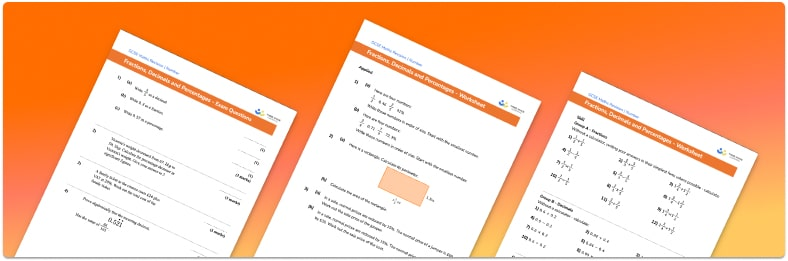# Fractions Decimals And Percentages Worksheets• Section 1 of the Fractions, Decimals and Percentages worksheet contains 48 skills-based questions, in 3 groups to support differentiation
• Section 2 contains 3 applied Fractions, Decimals and Percentages questions with a mix of worded problems and deeper problem solving questions
• Section 3 contains 4 foundation and higher level GCSE exam style Fractions, Decimals and Percentages questions
• Answers and a mark scheme for all Fractions, Decimals and Percentages questions
• Follows variation theory with plenty of opportunities for students to work independently at their own level
• All questions created by fully qualified expert secondary maths teachers
• Suitable for GCSE maths revision for AQA, OCR and Edexcel exam boards

• This field is for validation purposes and should be left unchanged.

You can unsubscribe at any time (each email we send will contain an easy way to unsubscribe). To find out more about how we use your data, see our privacy policy.

### Fractions decimals and percentages at a glance

Fractions, decimals and percentages (FDP) are all ways of representing parts of a whole. We can find equivalent percentages, fractions and decimals throughout the number system. These represent the same proportion of a whole, for example 50% = one half = 0.5.

To convert from a percentage to a decimal number, we divide the percentage by 100 and to convert from a decimal back to a percentage we multiply by 100.

When converting fractions to percentages we can find an equivalent fraction with a denominator of 100, this will mean that the numerator will then be the percentage (since percent means out of 100).

When converting fractions to decimals we can divide the numerator by the denominator, giving us the equivalent decimal.

When converting a decimal to a fraction, the numerator will be the numbers after the decimal point and the denominator will depend on the amount of numbers after the decimal point. For example if there is one decimal place (tenths), the denominator will be 10, if there are two decimal places (hundredths) it will be 100 and so on. We are often asked to give fractions in their simplest form so we should try and simplify any fraction we find.

We can calculate with fractions and decimals by adding, subtracting, multiplying and dividing them. We can also find a fraction or a percentage of an amount.

Looking forward, students can then progress to additional number worksheets, for example anorFor more teaching and learning support on Number our GCSE maths lessons provide step by step support for all GCSE maths concepts.

## Do you have KS4 students who need more focused attention to succeed at GCSE?There will be students in your class who require individual attention to help them succeed in their maths GCSEs. In a class of 30, it’s not always easy to provide.

Help your students feel confident with exam-style questions and the strategies they’ll need to answer them correctly with our dedicated GCSE maths revision programme.

Lessons are selected to provide support where each student needs it most, and specially-trained GCSE maths tutors adapt the pitch and pace of each lesson. This ensures a personalised revision programme that raises grades and boosts confidence.

Find out more